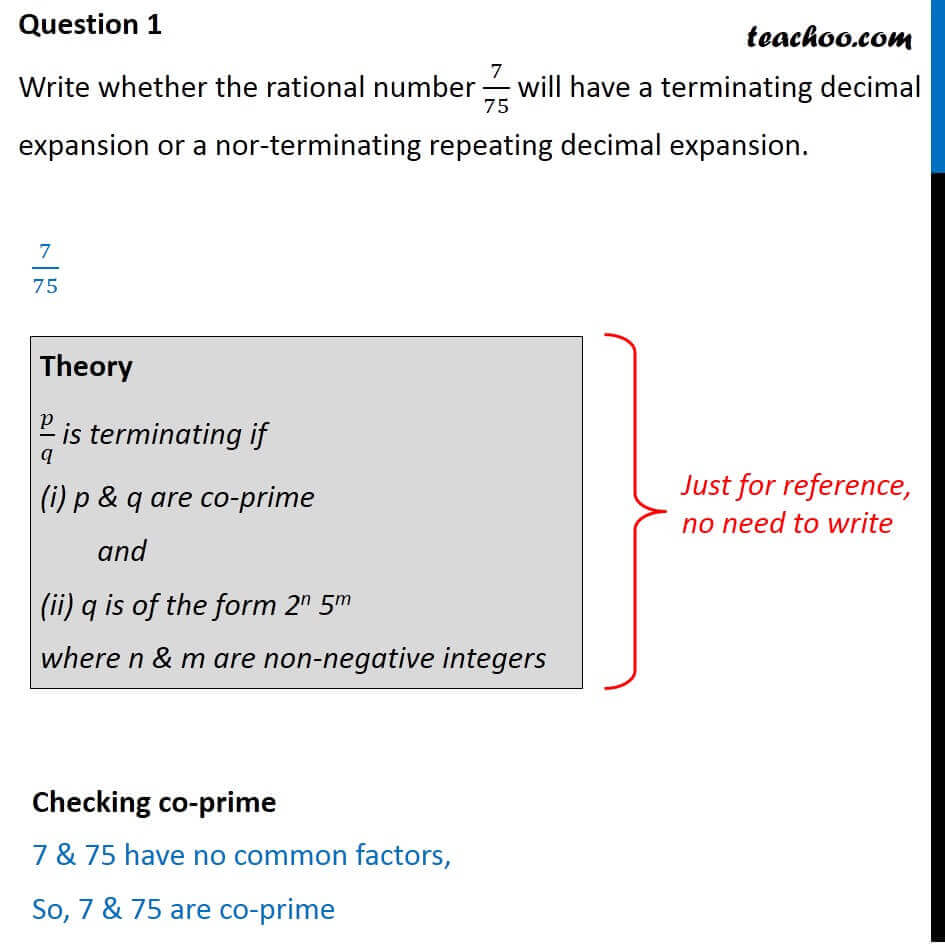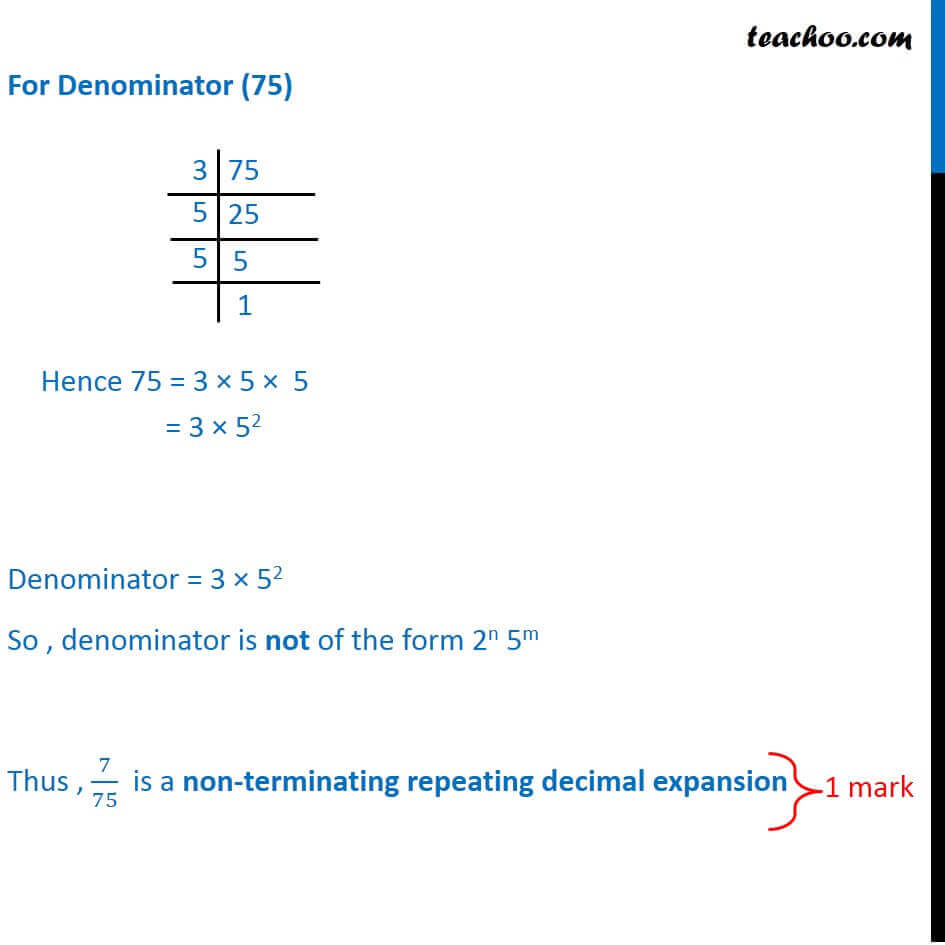### Write whether the rational number 7/75 will have a terminating decimal expansion or a non-terminating repeating decimal expansion.

This is a question of CBSE Sample Paper - Class 10 - 2017/18.1. Class 10
2. Solutions of Sample Papers for Class 10 Boards
3. CBSE Class 10 Sample Paper for 2018 Boards

Transcript

Question 1 Write whether the rational number 7/75 will have a terminating decimal expansion or a nor-terminating repeating decimal expansion. Theory / is terminating if (i) p & q are co-prime and (ii) q is of the form 2n 5m where n & m are non-negative integers 7/75 Checking co-prime 7 & 75 have no common factors, So, 7 & 75 are co-prime For Denominator (75) Denominator = 3 52 So , denominator is not of the form 2n 5m Thus , 7/75 is a non-terminating repeating decimal expansion Hence 75 = 3 5 5 = 3 52